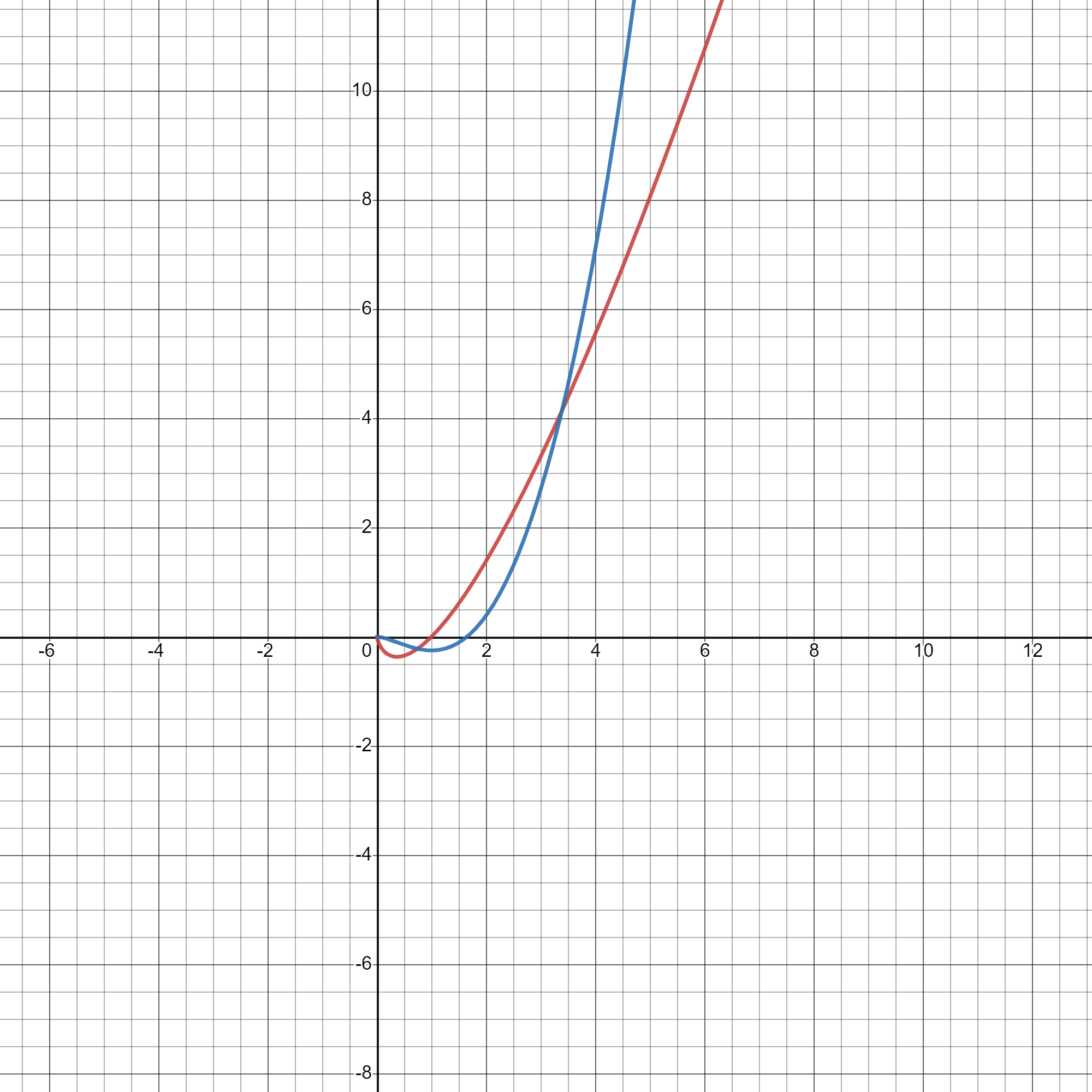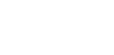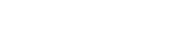# Category: Indefinite integrals## Integral of xln(x)

In this post I am going to explain step-by-step how to integrate the function xln(x). We can solve it by using integration by parts (click here if you want to… Read more Integral of xln(x)## Integral of sec²(x)|Two ways

Hi everyone! I know it’s been a really long time since my last post, but lessons during COVID-19 really had me busy and stressed out… but here I am again!… Read more Integral of sec²(x)|Two ways## Integral of ln²(x)

In this post I will show you how to find the anti-derivative of the function f(x)=ln²(x). We are going to use integration by parts: Now we can write u times… Read more Integral of ln²(x)## Integral with summations inside

I am a big fan of blackpenredpen. This guy posts videos on Youtube about calculus and more. Some days ago I received a notification and this is what the thumbnail… Read more Integral with summations inside## Integral of e^-x^t using Feynman’s technique

Wouldn’t it be nice to generalize the Gaussian integral to any exponent of –x? This is the famous integral: Since this is an even function, it can be written as… Read more Integral of e^-x^t using Feynman’s technique## Arc length formula

Today we are going to find the formula that allows us to calculate the arc length given a function f(x). This is quite intuitive, so you should have no problem!… Read more Arc length formula## Integral of e^(ax)*sin(x)

In this post we are going to integrate this seemingly hard integral, which in my opinion is actually very interesting! When we have a product of a trigonometric and a… Read more Integral of e^(ax)*sin(x)## Integral of sec²(x)/exp(tan(x))

Although it may look a bit intimidating at first, I can guarantee you this is actually a very easy integral! But what integration technique do you think will be useful?… Read more Integral of sec²(x)/exp(tan(x))

{{#pages}} {{/pages}}
%%footer%%